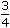# Aptitude - Stocks and Shares - Discussion

Discussion Forum : Stocks and Shares - General Questions (Q.No. 3)
3.
Which is better investment: 11% stock at 143  or  9% stock at 117?
11% stock at 143
 9 3 % stock at 117 4
Both are equally good
Cannot be compared, as the total amount of investment is not given.
Explanation:

Let investment in each case be Rs. (143 x 117).

 Income in 1st case = Rs.11 x 143 x 117= Rs. 1287. 143

 Income in 2nd case = Rs.39 x 143 x 117= Rs. 1394.25 4 x 117

 Clearly, 9 3 % stock at 117 is better. 4

Discussion:
27 comments Page 1 of 3.

Anirudh said:   1 year ago
They have assumed that the face value of both stocks is Rs 100. One more assumption is that only one share has been bought.

Akash said:   2 years ago
We need to assume that face value for both investment is same.

Akash said:   2 years ago
We need to assume that face value for both investment is same.

GAURAV said:   4 years ago
How investment is 143*117?
(2)

Abhilas said:   6 years ago
There is another efficient way.

For 117 in the first case (11/143)*117=9,
For 117 in the second case 9+(given).
Clearly second wins.

Raj said:   6 years ago
11% stock at 143 yields 25.75 (less profit).

9% stock at 117 yields 30.12 (more profit) <= preferable.

Shipra said:   6 years ago
Why answer is 2nd type of stock?

According to me,
1st case. 143*11%=15.73,
15.73/143*117=12.87,
2ndcase. 117*9.75%= 11.40.
So rate of interest is more in case 1st.

Santu said:   7 years ago
Let us assume face value of stock is Rs.100.

11% stock at 143 means:
11% = dividend rate which can be calculated on face value.i.e. dividend = 11% on 100 = Rs.11.
143 = market value of stock at which we can buy or sell the stock.

Here, we have to calculate the interest rate as we actually receive Rs.11 on investment of Rs.143 i.e. market value, the rate at which may be bought the stock.

Therefore, in 1st case interest rate = Rs.11/Rs.143 * 100=7.69%.
Similarly in 2nd case interest rate= Rs.9.75/Rs.117 * 100=8.33%.
As the interest is more in case 2.
The better investment is case 2.
(7)

ANNE said:   7 years ago
As 143 and 117 is not a facevalue, its only market vallue. And we have to calculate the rates on facevalue only, hence in both the cases we take the respective market value.

Vinay shankar said:   7 years ago
@ALL.

I think the term percent is misleading here.

As percent simply means out of hundred. So in my view, 13% means 13 out of 100 and it is solved for 13 out of 143.